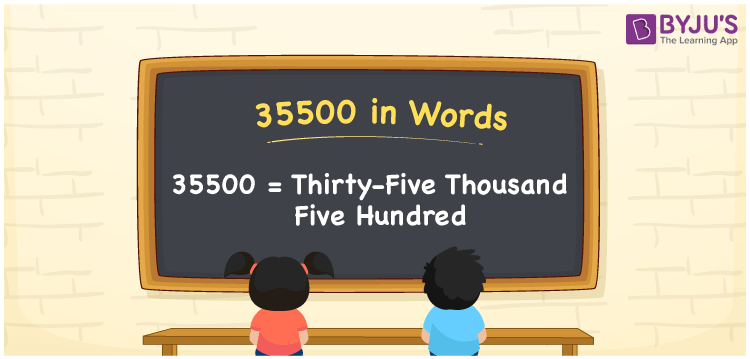# 35500 in words

35500 in words can be written as Thirty-five thousand five hundred . The article of 35500 in words will provide students with a clear knowledge of the concept of counting numbers. If Rs. 35500 is spent on buying new clothes, then you can say that “I spent Thirty-five thousand five hundred  Rupees in buying new clothes”. The  concept of converting 35500 into words using the English alphabet is discussed here. Students will be able to convert numbers in words with the help of the resources available at BYJU’S. 35500 can be read as “Thirty-five thousand five hundred ” in English.

 35500 in words Thirty-five thousand five hundred Thirty-five thousand five hundred  in Numbers 35500

## 35500 in English Words## How to Write 35500 in Words?

In this section you will learn in detail about conversion of 35500 into words using the place value chart. Five digits are present in 35500. The place value chart of 35500 can be understood easily using the table below.

 Ten Thousands Thousands Hundreds Tens Ones 3 5 5 0 0

The expanded form of the number 35500 is given below:

3 x Ten Thousand + 5 × Thousand + 5 × Hundred + 0 × Ten + 0 × One

= 3 x 10000 + 5 × 1000  + 5 × 100 + 0 × 10 + 0 × 1

= 30000 + 5000 + 500

= 35500

= Thirty-five thousand five hundred

Hence, 35500 in words is written as Thirty-five thousand five hundred .

35500 is a natural number that precedes 35501 and succeeds 35499.

35500 in words – Thirty-five thousand five hundred

Is 35500 an odd number? – No

Is 35500 an even number? – Yes

Is 35500 a perfect square number? – No

Is 35500 a perfect cube number? – No

Is 35500 a prime number? – No

Is 35500 a composite number? – Yes

## Frequently Asked Questions on 35500 in Words

Q1

### Write 35500 in words.

35500 can be written as “Thirty-five thousand five hundred” in words.
Q2

### Is 35500 an odd or even number?

35500 is an even number because it is divisible by 2.
35500/2 = 17750
Q3

### Write Thirty-five thousand five hundred in numbers.

Thirty-five thousand five hundred can be written in numbers as 35500.